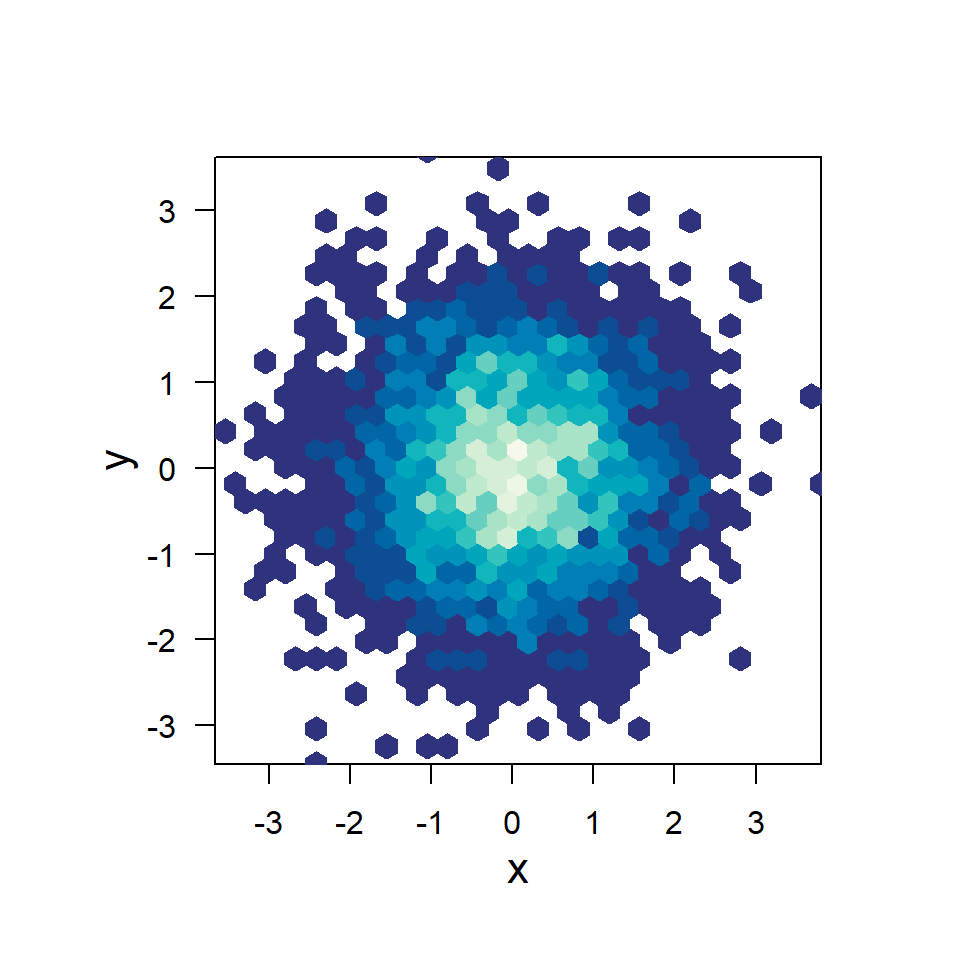# Hexbin chart in R

Edzer Pebesma

## The `hexbin` package

In base R it is possible to create a hexbin chart making use of the `hexbin` package, which contains a function of the same name to create a hexbin object that can be plotted.

``````# install.packages("hexbin")
library(hexbin)

# Data
set.seed(1)
x <- rnorm(5000)
y <- rnorm(5000)

hex <- hexbin(x, y)
plot(hex)``````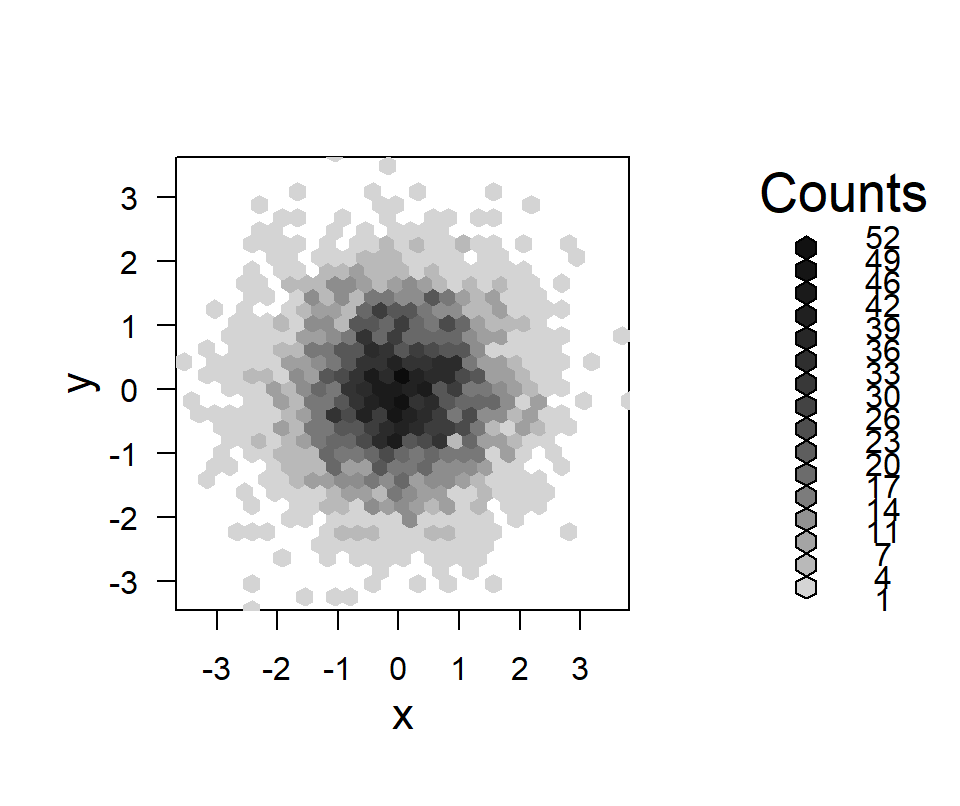Number of bins

The `xbins` argument controls the number of bins. The default value is 30.

``````# install.packages("hexbin")
library(hexbin)

# Data
set.seed(1)
x <- rnorm(5000)
y <- rnorm(5000)

hex <- hexbin(x, y, xbins = 20)
plot(hex)``````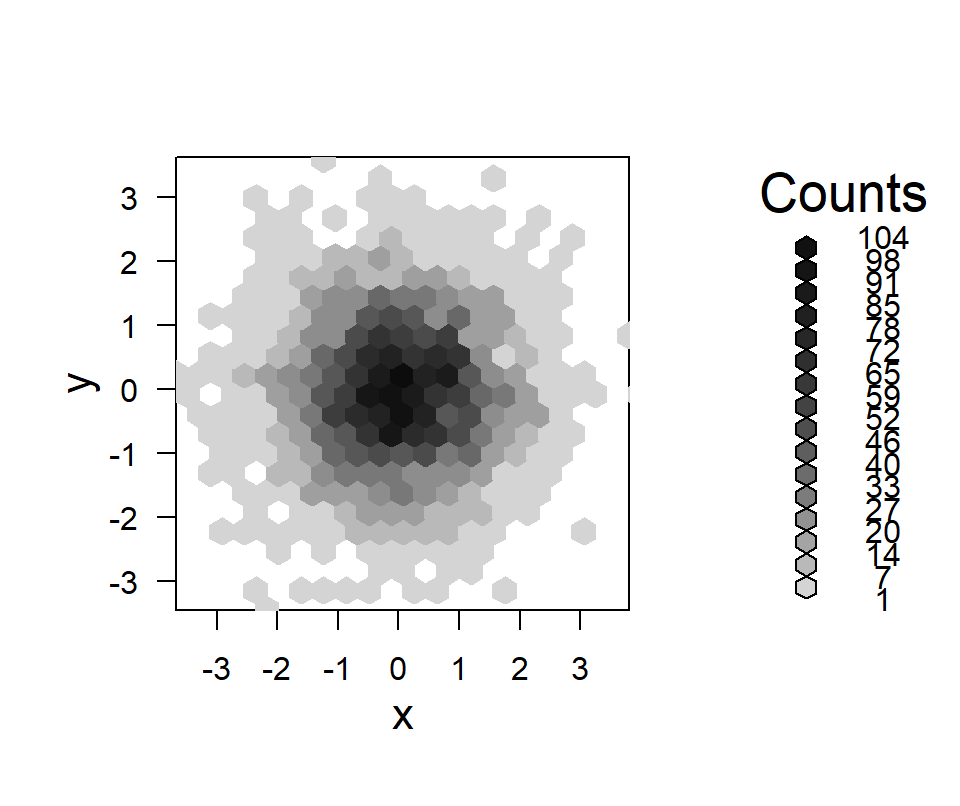## Color customization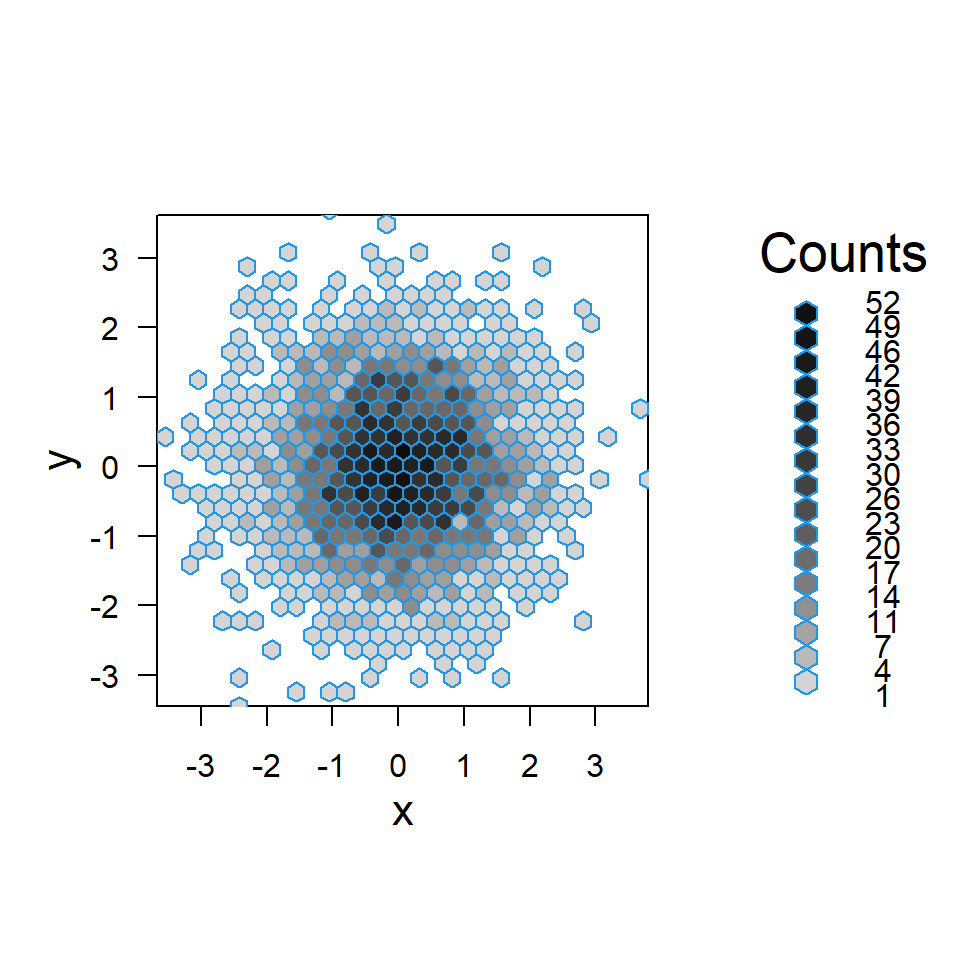Border color

The border color of the hexagons can be customized with the `boder` argument.

``````# install.packages("hexbin")
library(hexbin)

# Data
set.seed(1)
x <- rnorm(5000)
y <- rnorm(5000)

hex <- hexbin(x, y)
plot(hex, border = 4)``````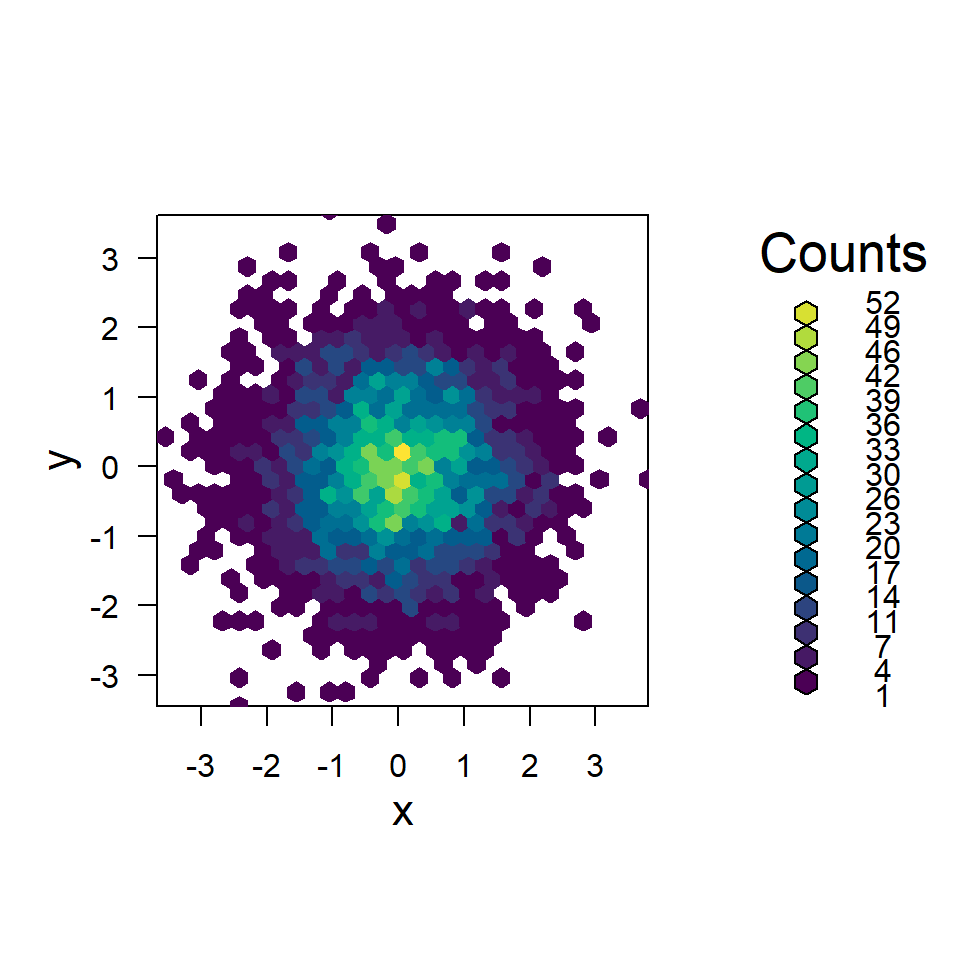Color palette

The default gray color palette can be customized passing a color ramp palette to the `colramp` argument. In the following example we are passing 12 colors of the viridis palette with the `hcl.colors` function.

``````# install.packages("hexbin")
library(hexbin)

# Data
set.seed(1)
x <- rnorm(5000)
y <- rnorm(5000)

hex <- hexbin(x, y)
plot(hex, colramp = colorRampPalette(hcl.colors(12)))``````

## Legend

Legend width

The `legend` argument allows customizing the width of the default legend. However, if the value is too small the legend will be croped. Default value is 1.2.

``````# install.packages("hexbin")
library(hexbin)

# Data
set.seed(1)
x <- rnorm(5000)
y <- rnorm(5000)

hex <- hexbin(x, y)
plot(hex, legend = 0.9)``````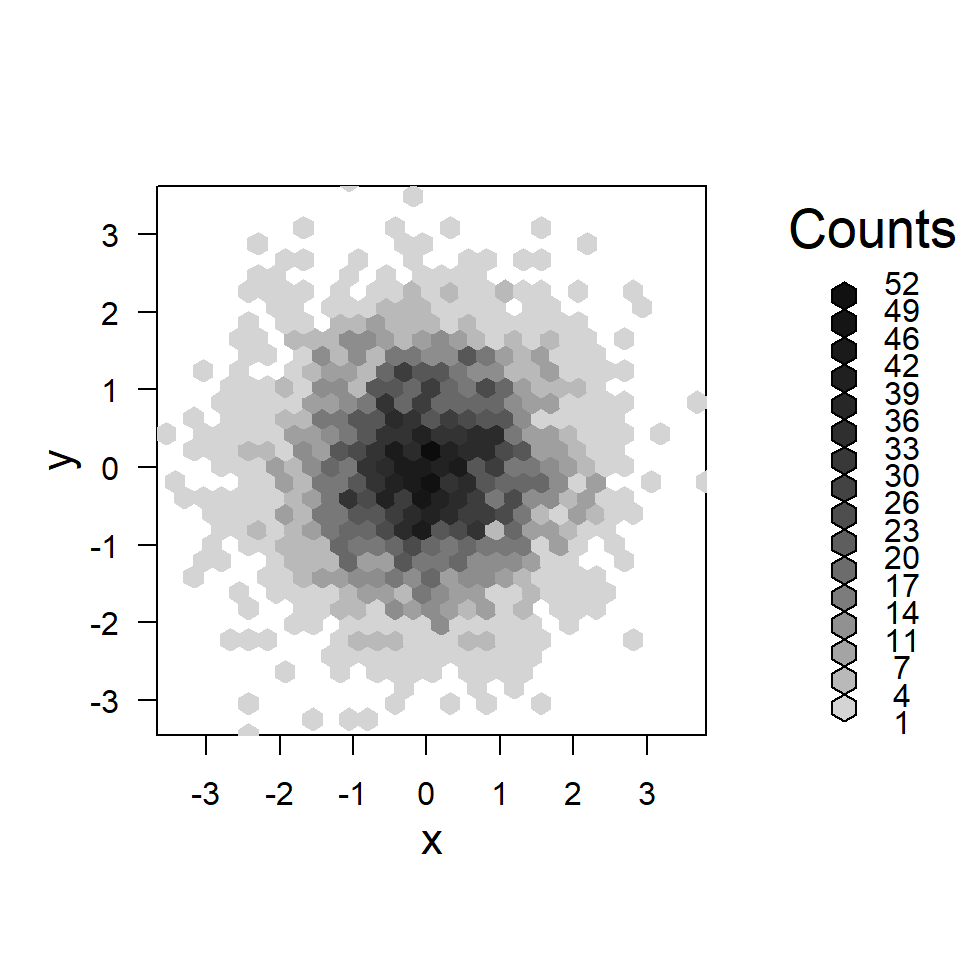Text size of the legend

You can also change the text size of the legend with `lcex`.

``````# install.packages("hexbin")
library(hexbin)

# Data
set.seed(1)
x <- rnorm(5000)
y <- rnorm(5000)

hex <- hexbin(x, y)
plot(hex, lcex = 0.9)``````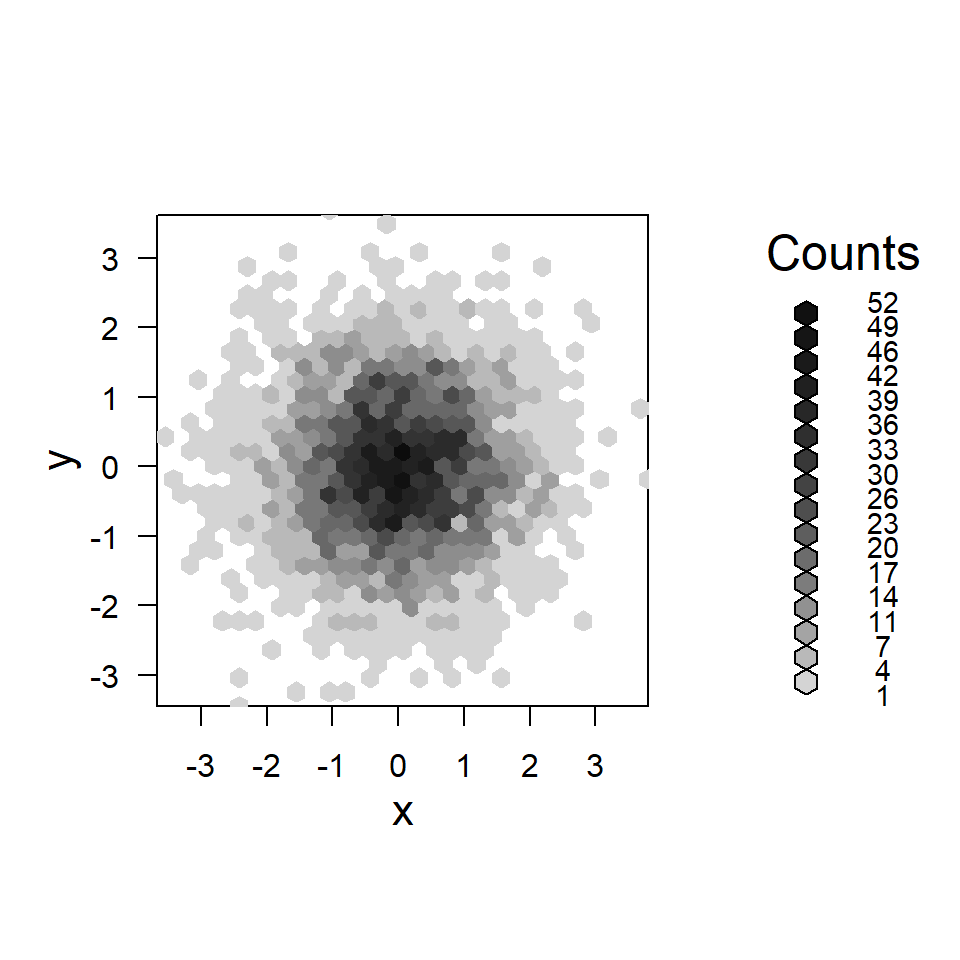Remove the legend

Finally, if you want to remove the legend set the `legend` argument to `FALSE`.

``````# install.packages("hexbin")
library(hexbin)

# Data
set.seed(1)
x <- rnorm(5000)
y <- rnorm(5000)

hex <- hexbin(x, y)
plot(hex, legend = FALSE,
colramp = colorRampPalette(hcl.colors(12, "GnBu")))``````